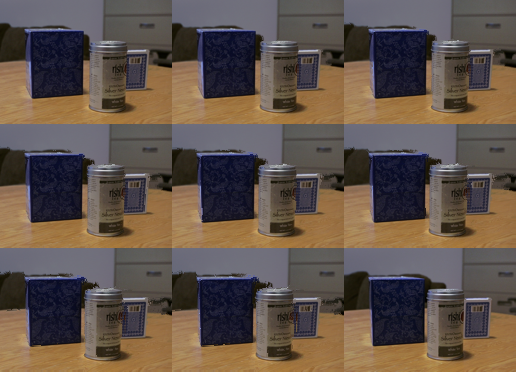光流的计算

• 前后两帧点的位移不大（泰勒展开）
• 外界光强保持恒定。
• 空间相关性，每个点的运动和他们的邻居相似（连续函数，泰勒展开）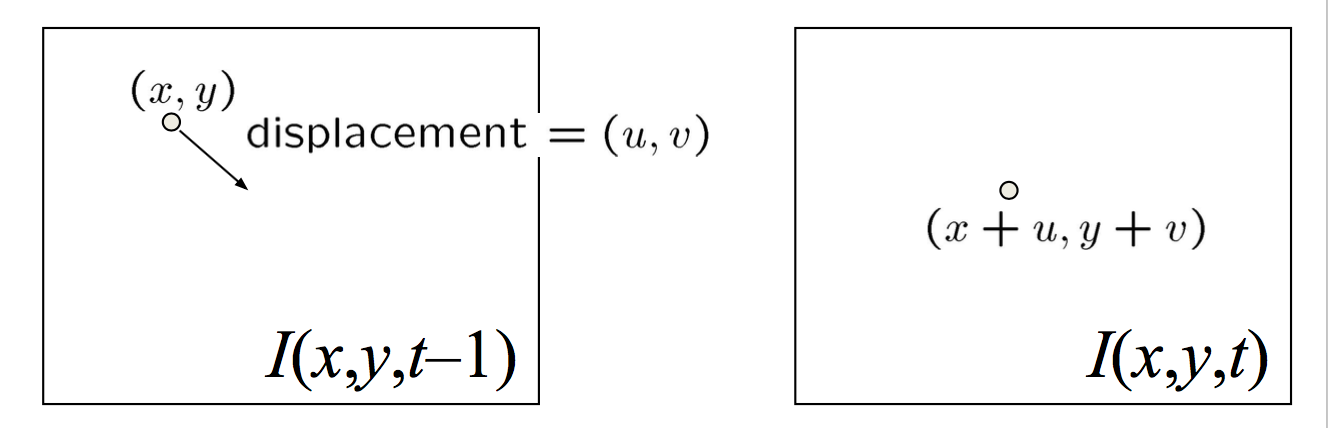L-K方法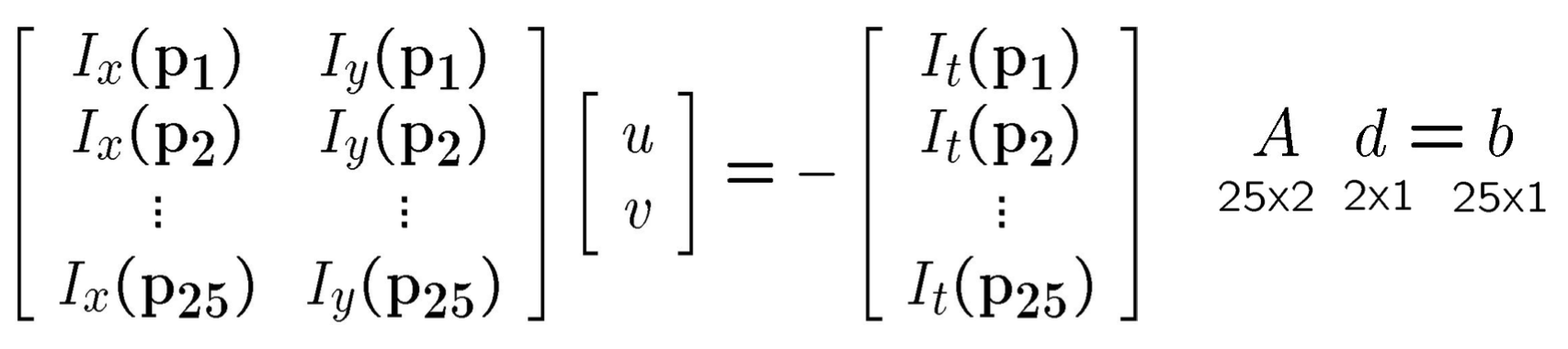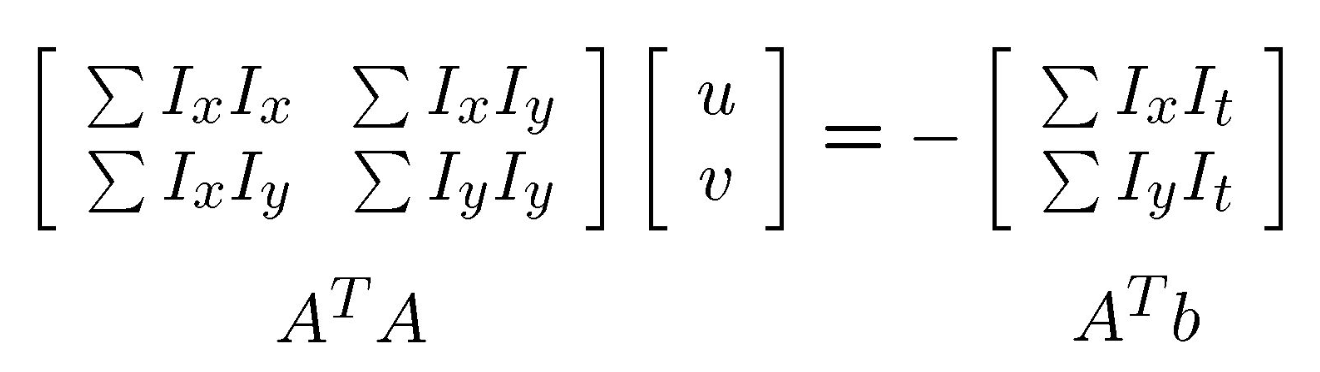• $\mathbf{A}^\dagger \mathbf{A}$是可逆的。
• $\mathbf{A}^\dagger \mathbf{A}$不应该太小（噪声）。这意味着它的特征值$\lambda_1$, $\lambda_2$不应该太小。
• $\mathbf{A}^\dagger \mathbf{A}$不应该是病态的（稳定性）。这意味着它的特征值$\lambda_1/\lambda_2$不应该太大。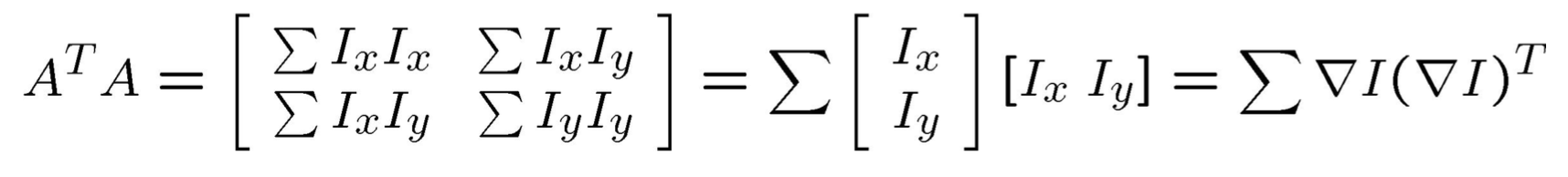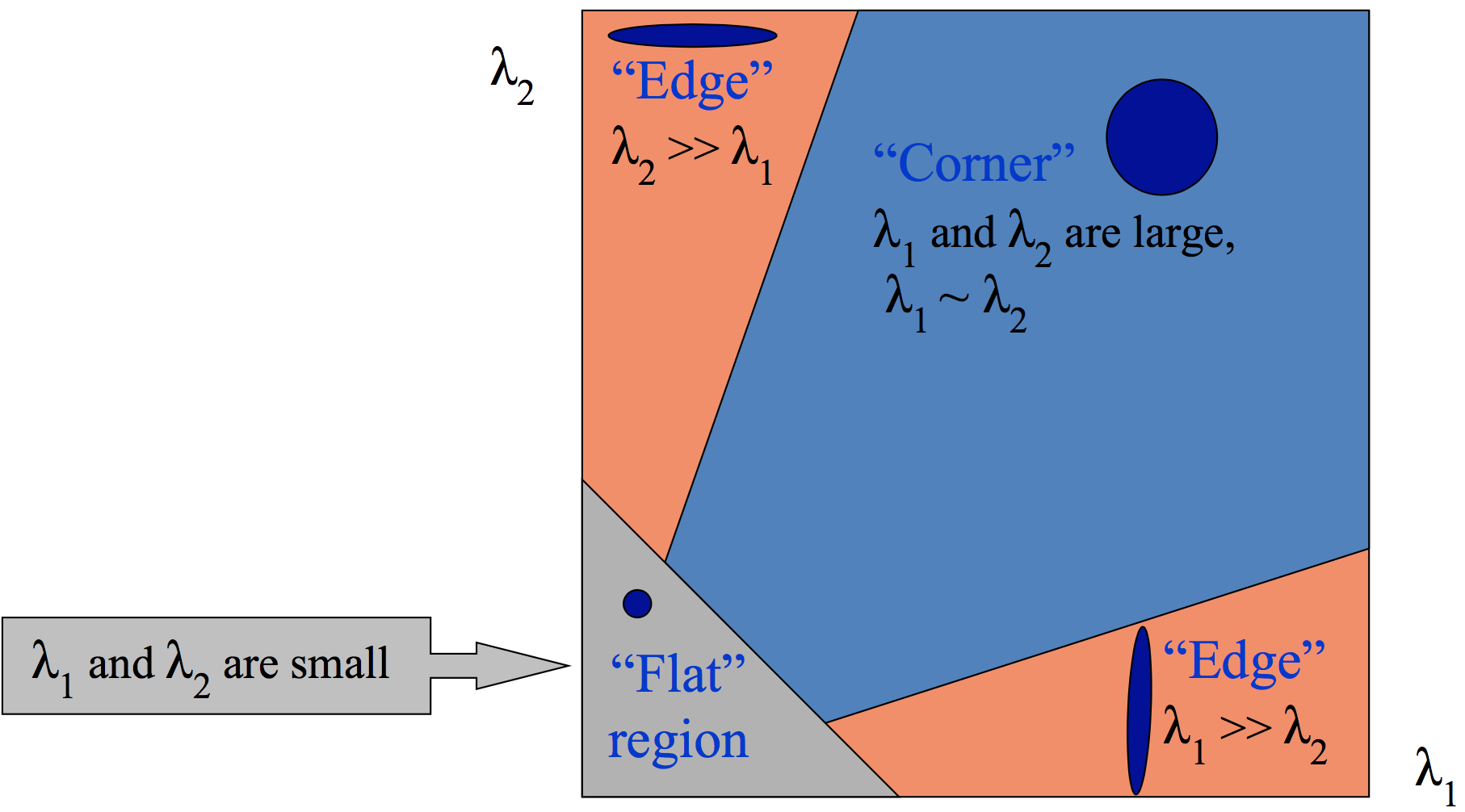金字塔方法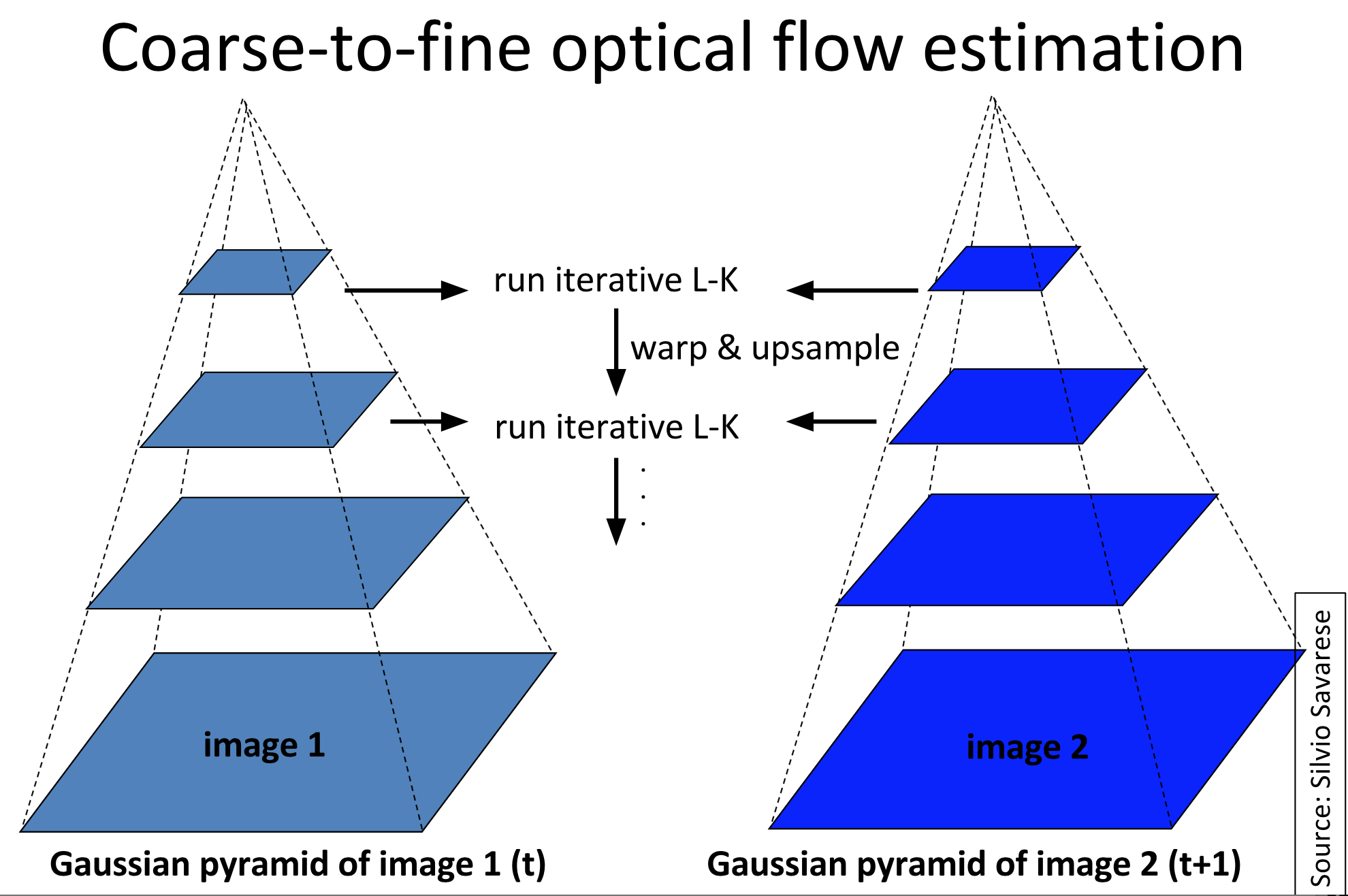作业：基于光流法的帧间插值

简单粗暴法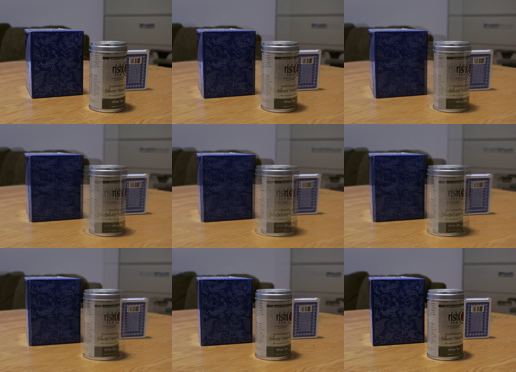基于光流法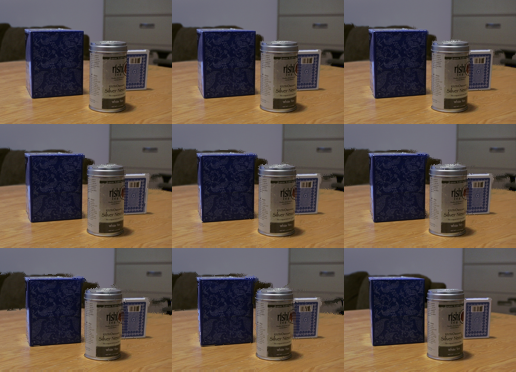改进